Courses

# Strings Civil Engineering (CE) Notes | EduRev

## Civil Engineering (CE) : Strings Civil Engineering (CE) Notes | EduRev

The document Strings Civil Engineering (CE) Notes | EduRev is a part of the Civil Engineering (CE) Course Civil Engineering SSC JE (Technical).
All you need of Civil Engineering (CE) at this link: Civil Engineering (CE)

Chapter 9

SPRINGS
Spring is a device, in which the material is arranged in such a way that it can undergo a considerable change, without getting permanently distorted.  A spring is used to absorb energy due to resilience which may be restored as and when required.  The quality of a spring is judged form the energy it can absorb. The spring which is capable of absorbing the greatest amount of energy for the given stress is the best one.

Stiffness of a Spring 

• The load required to produce a unit deflection in spring is called spring stiffness.

Types of Springs There are two types depending upon the type of resilience: 

• Bending spring (leaf spring) 
• Torsion spring (helical spring)

Leaf Springs

Central deflection d is given by,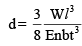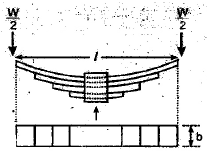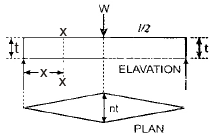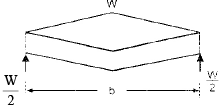Where,

l = Span of spring
t = thickness of plates
b = Width of plates
n = Number of plates
W = Load acting on the spring
E = Young's modulus

Closed coiled helical springs subjected to axial loading 

Deflection in the spring due to load W,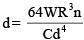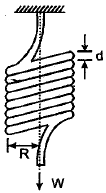• Stiffness of the spring,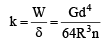Where, W = axial load n = no. of turns of spring d = diameter of the rod of the spring R = mean radius of the coil  G = modulus of rigidity for the spring material.

• Energy stored,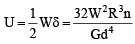Closely coiled helical spring subjected to axial twist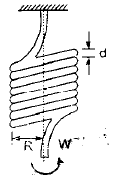• Total angle of bend = φ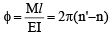where l = 2 pRn = 2pR ' n '

•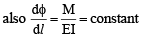Thus, the change in curvature or angle of bend per unit length, is constant, throughout the spring.

•  Energy stored =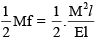Where, R = mean radius of the spring coil
n = no. of turns or coils
M = Moment or axial twist applied on the spring
R = decreased mean radius due to twist
n = increased no of turns due to twist
I = moment of inertia of spring rod section
E = Young's modulus

Open coiled helical spring subjected to axial load 

• In  this case load W will cause both twisting and bending of coils.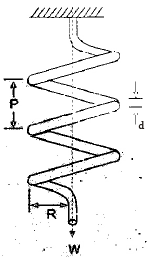• Deflection of the spring as a result as a result of axial load,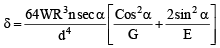Where,
d = Diameter of spring wire
R = Mean radius of spring coil
P = Pitch of the spring coil
n = no. of turns of coils
G = modulus of rigidity for spring material

W = axial load on the spring
a = angle of helix

E = Young's modulus 

• If we put µ = o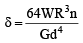= deflection of closed coiled spring.

SPRINGS IN SERIES AND PARALLEL
(i) Springs in series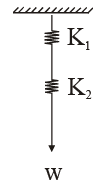• Total  extension = å (individual extensions)

d = d1 + d2 

• load applied (W) will be same on both the springs. 
• The equivalent stiffness is given by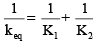where K1 & K2 are individual stiffness of the springs.

(ii) Springs in parallelBoth the springs have same extension.
δ = δ1 = δ2 

Load applied (W) is shared by the springs.
W = W1 + W2
The equivalent stiffness is given by,

Keq = K+ K2

Offer running on EduRev: Apply code STAYHOME200 to get INR 200 off on our premium plan EduRev Infinity!

## Civil Engineering SSC JE (Technical)

113 docs|50 tests

,

,

,

,

,

,

,

,

,

,

,

,

,

,

,

,

,

,

,

,

,

;